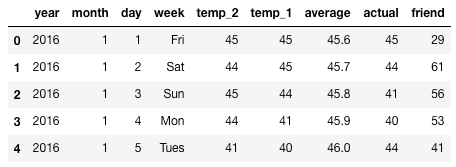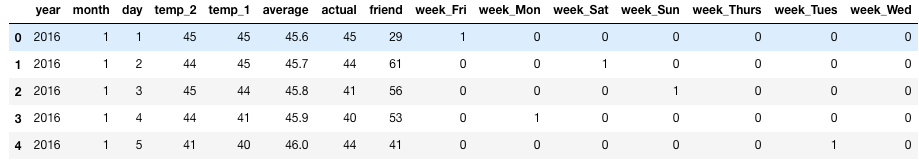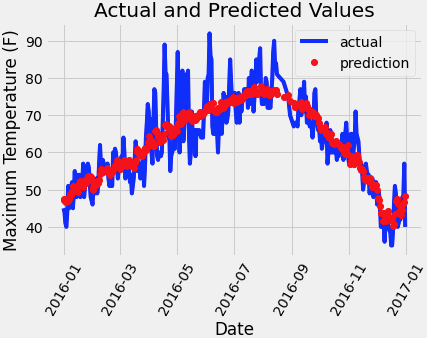## pytorch回归模型_pytorch回归预测_Andy_shenzl的博客-程序员宅基地

### 数据简介

``````features = pd.read_csv('temps.csv')
``````• year,moth,day,week分别表示的具体的时间
• temp_2：前天的最高温度值
• temp_1：昨天的最高温度值
• average：在历史中，每年这一天的平均最高温度值
• actual：这就是我们的标签值了，当天的真实最高温度
• friend：这一列可能是凑热闹的，你的朋友猜测的可能值，咱们不管它就好了

``````# 处理时间数据
import datetime

# 分别得到年，月，日
years = features['year']
months = features['month']
days = features['day']

# datetime格式
dates = [str(int(year)) + '-' + str(int(month)) + '-' + str(int(day)) for year, month, day in zip(years, months, days)]
dates = [datetime.datetime.strptime(date, '%Y-%m-%d') for date in dates]

dates[:5]
``````

[datetime.datetime(2016, 1, 1, 0, 0),
datetime.datetime(2016, 1, 2, 0, 0),
datetime.datetime(2016, 1, 3, 0, 0),
datetime.datetime(2016, 1, 4, 0, 0),
datetime.datetime(2016, 1, 5, 0, 0)]

``````# 独热编码
features = pd.get_dummies(features)
````````````# 标签
labels = np.array(features['actual'])

# 在特征中去掉标签
features= features.drop('actual', axis = 1)

# 名字单独保存一下，以备后患
feature_list = list(features.columns)

# 转换成合适的格式
features = np.array(features)

from sklearn import preprocessing
input_features = preprocessing.StandardScaler().fit_transform(features)
``````

#### 构建预测网络-基础版

``````#先把数据转化成tensor格式

x = torch.tensor(input_features, dtype = float)

y = torch.tensor(labels, dtype = float)

# 权重参数初始化
weights = torch.randn((14, 128), dtype = float, requires_grad = True)
biases = torch.randn(128, dtype = float, requires_grad = True)
weights2 = torch.randn((128, 1), dtype = float, requires_grad = True)
biases2 = torch.randn(1, dtype = float, requires_grad = True)

learning_rate = 0.001
losses = []
``````

``````for i in range(1000):
# 计算隐层
hidden = x.mm(weights) + biases
# 加入激活函数
hidden = torch.relu(hidden)
# 预测结果
predictions = hidden.mm(weights2) + biases2
# 通计算损失
loss = torch.mean((predictions - y) ** 2)
losses.append(loss.data.numpy())

# 打印损失值
if i % 100 == 0:
print('loss:', loss)
#返向传播计算
loss.backward()

#更新参数,加上负的梯度

# 每次迭代都得记得清空

``````

#### 简单版

``````
#参数设置
input_size = input_features.shape
hidden_size = 128
output_size = 1
batch_size = 16
#整体模型架构
my_nn = torch.nn.Sequential(
torch.nn.Linear(input_size, hidden_size),
torch.nn.Sigmoid(),
torch.nn.Linear(hidden_size, output_size),
)
#损失函数
cost = torch.nn.MSELoss(reduction='mean')
#参数
optimizer = torch.optim.Adam(my_nn.parameters(), lr = 0.001)
``````

``````# 训练网络
losses = []
for i in range(1000):
batch_loss = []
# MINI-Batch方法来进行训练
for start in range(0, len(input_features), batch_size):
end = start + batch_size if start + batch_size < len(input_features) else len(input_features)
xx = torch.tensor(input_features[start:end], dtype = torch.float, requires_grad = True)
yy = torch.tensor(labels[start:end], dtype = torch.float, requires_grad = True)
prediction = my_nn(xx)
loss = cost(prediction, yy)
loss.backward(retain_graph=True)
optimizer.step()
batch_loss.append(loss.data.numpy())

# 打印损失
if i % 100==0:
losses.append(np.mean(batch_loss))
print(i, np.mean(batch_loss))
``````

0 3950.7627
100 37.9201
200 35.654438
300 35.278366
400 35.116814
500 34.986076
600 34.868954
700 34.75414
800 34.637356
900 34.516705

``````x = torch.tensor(input_features, dtype = torch.float)
predict = my_nn(x).data.numpy()
``````### mysql_query失败_php中mysqli_query失败_weixin_39818631的博客-程序员宅基地

PHP mysqli_query() 函数定义和用法(推荐学习：PHP视频教程)mysqli_query() 函数执行某个针对数据库的查询。语法mysqli_query(connection,query,resultmode);参数说明：connection：必填。规定要使用的 MySQL 连接。query：必填，规定查询字符串。resultmode：可选。一个常量。可以是下列值中的任意一个：MY...

### vue获取接口id_05vue2.0-vue中_接口的调用_weixin_39675215的博客-程序员宅基地

promise主要解决异步深层嵌套的问题promise 提供了简洁的API 使得异步操作更加容易/*1. Promise基本使用我们使用new来构建一个Promise Promise的构造函数接收一个参数，是函数，并且传入两个参数： resolve，reject， 分别表示异步操作执行成功后的回调函数和异步操作执行失败后的回调函数*/var p = new Promise(...

### Abp项目（.net） 部署到服务端IIS 无法正常打开页面运行问题_iis部署web项目页面出不来_小丫头呀的博客-程序员宅基地

IIS　-> 应用程序池 -> 具体应用 -> 右键选择高级设置 -> 找到进程模型 -> 标识 -> 选择自定义账户 -> 输入administrator 账号密码 ，重启应用程序解决。注意：部署到iis，是要安装.net 运行时的。服务端IIS对调用exe程序有权限限制，没有正常启动插件，需要给调用插件的应用程序池赋予足够权限。5.1 在iis中找到建立的网站，打开应用程序池 ，把CLR 改成无托管代码，防止被回收。5.6 重启应用程序，选上最上一级，点击重启。

### c语言 中文版下载32位,C-Free5.0免费版_weixin_39629631的博客-程序员宅基地

C-Free是一款专业的C语言编译器，软件成了C/C++代码解析器，拥有实时解析代码的功能，有从事C语言开发的用户不要错过，小编为大家带来的是中文专业版本，更适合开发用户使用。C-Free提供了对目前业界主流C/C++编译器的支持，你可以在C-Free中轻松切换编译器。可定制的快捷键、外部工具以及外部帮助文档，使你在编写代码时得心应手。完善的工程/工程组管理使你能够方便的管理自己的代码。C-Fre...